Does this quadrilateral have only one pair of equal opposite angles?

This Math quiz is called 'Quadrilaterals (Properties)' and it has been written by teachers to help you if you are studying the subject at high school. Playing educational quizzes is a user-friendly way to learn if you are in the 9th or 10th grade - aged 14 to 16.

It costs only \$12.50 per month to play this quiz and over 3,500 others that help you with your school work. You can subscribe on the page at Join Us

Quadrilaterals belong to the polygon family, and are so named as they have four sides (this comes from Latin, with QUAD meaning 4, and LATERAL meaning sides). See how well you know the properties of quadrilaterals in this high school Math quiz.

Quadrilaterals, like triangles, appear just about everywhere, and so it is important to have a good understanding of their properties. Starting at a very general level, the sum of the interior angles will add up to 360o. Can you remember why this is so? Draw in a diagonal, and it will form 2 triangles. The sum of the angles in a triangle is 180o, so the sum of two triangles will be 360o.

Once you start adding on other properties, such as a pair of parallel sides, opposite sides are equal length, diagonals bisect each other, then you create special quadrilaterals, that each have their own name.

You will need to know what makes a rhombus, what is special about a trapezium, or a parallelogram (think parallel bars in gymnastics), or a kite.

We often think of a square as a special case of a rectangle – they both have 4 right-angles, a rectangle has 2 pairs of equal sides while a square has all sides equal. But a square could also be considered as a special case of a rhombus – a rhombus is a parallelogram with all sides equal, and a square is a rhombus with all angles equal.

Quadrilaterals whose diagonals intersect at 90o are particularly important when it comes to Pythagoras and Trigonometry, can you work out why?

Question 1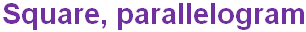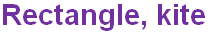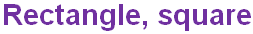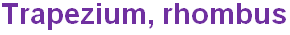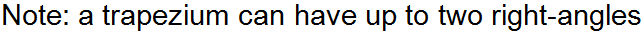Question 2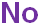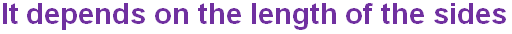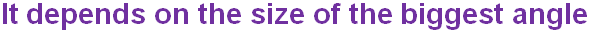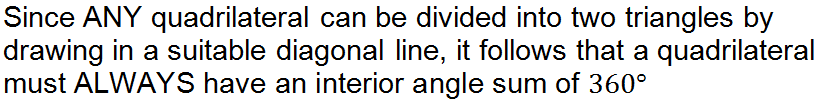Question 3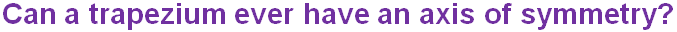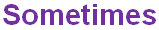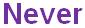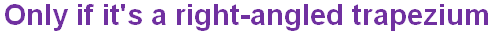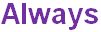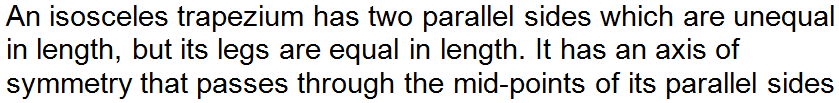Question 4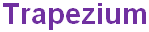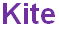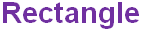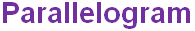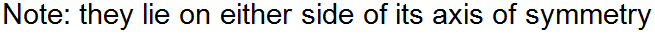Question 5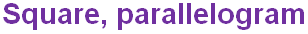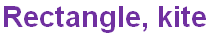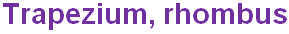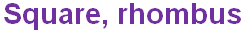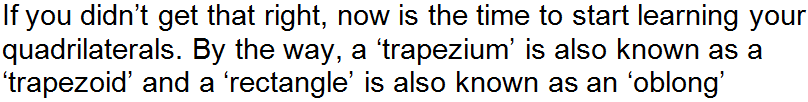Question 6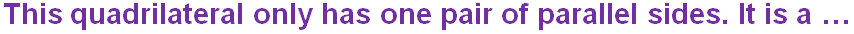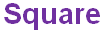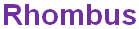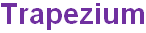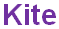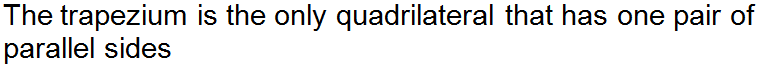Question 7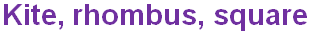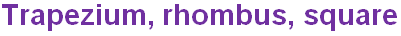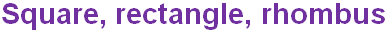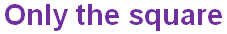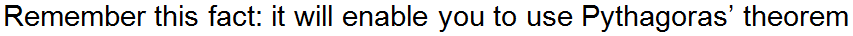Question 8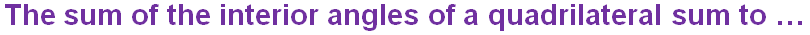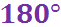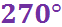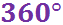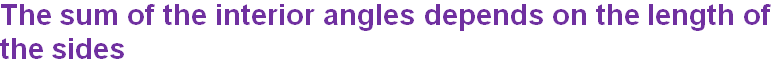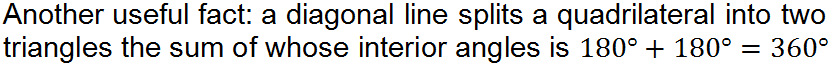Question 9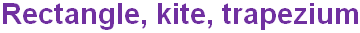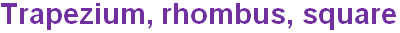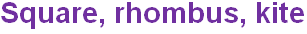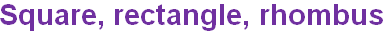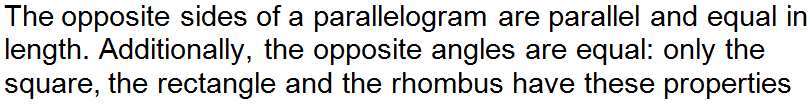Question 10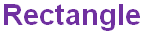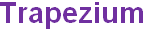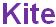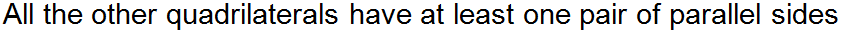Author:  Frank Evans